#### 期刊菜单

Stability Analysis of Functionally Graded Circular Plates Considering Pre-Buckling Coupling Deformation
DOI: 10.12677/IJM.2021.101002, PDF , HTML, XML, 下载: 306  浏览: 589  国家自然科学基金支持

Abstract: The inhomogeneity of functionally graded materials (FGM) structure comes from the gradient change of material components along a certain direction, so there will be stretch bending coupling effect. As long as the in-plane load is applied, deflection will occur, in the case of simply supported boundary conditions. Based on the classical plate theory, the nonlinear deformation and stability of FGM simply supported circular plates under mechanical and thermal loads are studied in this paper. Assuming that the material properties of FGM circular plate only change along the thickness direction, the equilibrium equation of FGM circular plate is derived by using the energy principle, and the governing equation including the influence of pre-buckling coupling deformation is obtained, which is solved by shooting method. The effects of external loads, pre-buckling coupling deformation and temperature dependence of material properties on the nonlinear deformation and stability of FGM circular plate are discussed.

1. 引言

FGM结构由于具有非均匀性，在简支边界条件下会引起前屈曲耦合变形，即只要面内载荷存在，就产生耦合挠度。这种情形下，FGM简支圆板的屈曲问题变得十分复杂，与均匀材料圆板有着显著的区别    。本文中屈曲是指面内载荷有微小变化就会引起圆板挠度的大幅度增加或减小，在其载荷–挠度曲线上出现一个“平台”   。杨帆和马连生  的研究结果表明，前屈曲耦合变形对FGM圆板的稳定性确实有一定影响。而过去的一些研究成果没有考虑前屈曲耦合变形，掉进了Quta和Leissa   所称的“陷阱”，这个观点也被很多研究人员的成果       所证实。

2. 基本方程

$P\left(z\right)=\left({P}_{c}-{P}_{m}\right){\left(\frac{h+2z}{2h}\right)}^{n}+{P}_{m}$ (1)

$P={P}_{0}\left({P}_{-1}{T}^{-1}+1+{P}_{1}T+{P}_{2}{T}^{2}+{P}_{3}{T}^{3}\right)$ (2)

$-\frac{\text{d}}{\text{d}z}\left(K\left(z\right)\frac{\text{d}T\left(z\right)}{\text{d}z}\right)=0$ (3)

$T\left(z\right)={T}_{m}+\left({T}_{c}-{T}_{m}\right){\int }_{-h/2}^{z}\frac{\text{d}z}{K\left(z\right)}/{\int }_{-h/2}^{h/2}\frac{\text{d}z}{K\left(z\right)}$ (4)

${U}_{r}\left(r,z\right)=U\left(r\right)-z{W}_{,r},{U}_{z}\left(r,z\right)=W\left(r\right)$ (5)

${\epsilon }_{r}={U}_{,r}+\frac{{\left({W}_{,r}\right)}^{2}}{2}-z{W}_{,rr}={\epsilon }_{r}^{0}+z{k}_{r},{\epsilon }_{\theta }=\frac{U}{r}-\frac{z{W}_{,r}}{r}={\epsilon }_{\theta }^{0}+z{k}_{\theta }$ (6)

${N}_{r}={A}_{11}\left(\frac{\partial U}{\partial r}+\nu \frac{U}{r}+\frac{1}{2}{\left(\frac{\partial W}{\partial r}\right)}^{2}\right)-{B}_{11}\left(\frac{{\partial }^{2}W}{\partial {r}^{2}}+\frac{\nu }{r}\frac{\partial W}{\partial r}\right)-{N}^{T}$ (7a)

${N}_{\theta }={A}_{11}\left(\nu \frac{\partial U}{\partial r}+\frac{U}{r}+\frac{\nu }{2}{\left(\frac{\partial W}{\partial r}\right)}^{2}\right)-{B}_{11}\left(\nu \frac{{\partial }^{2}W}{\partial {r}^{2}}+\frac{1}{r}\frac{\partial W}{\partial r}\right)-{N}^{T}$ (7b)

${M}_{r}={B}_{11}\left(\frac{\partial U}{\partial r}+\nu \frac{U}{r}+\frac{1}{2}{\left(\frac{\partial W}{\partial r}\right)}^{2}\right)-{D}_{11}\left(\frac{{\partial }^{2}W}{\partial {r}^{2}}+\frac{\nu }{r}\frac{\partial W}{\partial r}\right)-{M}^{T}$ (7c)

${M}_{\theta }={B}_{11}\left(\nu \frac{\partial U}{\partial r}+\frac{U}{r}+\frac{\nu }{2}{\left(\frac{\partial W}{\partial r}\right)}^{2}\right)-{D}_{11}\left(\nu \frac{{\partial }^{2}W}{\partial {r}^{2}}+\frac{1}{r}\frac{\partial W}{\partial r}\right)-{M}^{T}$ (7d)

$\left({A}_{11},{B}_{11},{D}_{11}\right)={\int }_{-h/2}^{h/2}{Q}_{11}\left(1,z,{z}^{2}\right)\text{d}z,{Q}_{11}=\frac{E\left(z\right)}{1-{\nu }^{2}}$ (8a)

$\left({N}^{T},{M}^{T}\right)={\int }_{-h/2}^{h/2}\frac{E\left(z\right)}{1-\nu }\alpha \left(z\right)T\left(z\right)\left(1,z\right)\text{d}z$ (8b)

$\frac{\text{d}}{\text{d}x}\left[\frac{1}{x}\frac{\text{d}}{\text{d}x}\left(xu\right)\right]+\left[\frac{\text{d}w}{\text{d}x}\frac{{\text{d}}^{2}w}{\text{d}{x}^{2}}+\frac{1-\nu }{2x}{\left(\frac{\text{d}w}{\text{d}x}\right)}^{2}\right]-{F}_{1}\frac{\text{d}}{\text{d}x}{\nabla }^{2}w=0$ (9a)

$\begin{array}{l}{\nabla }^{4}w-{F}_{2}\left[\nu \frac{\text{d}u}{\text{d}x}+\frac{u}{x}+\frac{\nu }{2}{\left(\frac{\text{d}w}{\text{d}x}\right)}^{2}\right]\frac{1}{x}\frac{\text{d}w}{\text{d}x}-{F}_{2}\left[\frac{\text{d}u}{\text{d}x}+\nu \frac{u}{x}+\frac{1}{2}{\left(\frac{\text{d}w}{\text{d}x}\right)}^{2}\right]\frac{{\text{d}}^{2}w}{\text{d}{x}^{2}}\\ +{F}_{3}\left[{\left(\frac{{\text{d}}^{2}w}{\text{d}{x}^{2}}\right)}^{2}+\frac{2\nu }{x}\frac{\text{d}w}{\text{d}x}\frac{{\text{d}}^{2}w}{\text{d}{x}^{2}}+\frac{1}{{x}^{2}}{\left(\frac{\text{d}w}{\text{d}x}\right)}^{2}\right]+\stackrel{¯}{N}{\nabla }^{2}w=0\end{array}$ (9b)

$u=\frac{\text{d}w}{\text{d}x}=0,\underset{x\to 0}{\mathrm{lim}}\left(\frac{{\text{d}}^{3}w}{\text{d}{x}^{3}}+\frac{1}{x}\frac{{\text{d}}^{2}w}{\text{d}{x}^{2}}\right)=0$ (10)

$u=w=0,{F}_{4}\left[\frac{\text{d}u}{\text{d}x}+\frac{1}{2}{\left(\frac{\text{d}w}{\text{d}x}\right)}^{2}\right]-\left(\frac{{\text{d}}^{2}w}{\text{d}{x}^{2}}+\frac{\nu }{x}\frac{\text{d}w}{\text{d}x}\right)-\stackrel{¯}{M}=0$ (11)

$\begin{array}{l}x=\frac{r}{b},w=\frac{W}{h},u=\frac{Ub}{{h}^{2}},{F}_{1}=\frac{{F}_{3}}{{F}_{2}},{F}_{2}=\frac{{A}_{11}{h}^{2}}{\Omega },{F}_{3}=\frac{{B}_{11}h}{\Omega },{F}_{4}=\frac{{B}_{11}h}{{D}_{11}},\\ \stackrel{¯}{N}=\frac{{N}^{T}{b}^{2}}{\Omega },\stackrel{¯}{M}=\frac{{M}^{T}{b}^{2}}{{D}_{11}h},\Omega ={D}_{11}-\frac{{B}_{11}^{2}}{{A}_{11}},{F}_{p}=\frac{p{b}^{2}}{{A}_{11}{h}^{2}}\end{array}$

$u=\stackrel{¯}{u}+\Delta u,w=\stackrel{¯}{w}+\Delta w$ (12)

$\begin{array}{l}{\nabla }^{4}\Delta w-{F}_{2}\left[\nu \frac{\text{d}\Delta u}{\text{d}x}+\frac{\Delta u}{x}+\nu \frac{\text{d}\Delta w}{\text{d}x}\frac{\text{d}\stackrel{¯}{w}}{\text{d}x}\right]\frac{1}{x}\frac{\text{d}\stackrel{¯}{w}}{\text{d}x}-{F}_{2}\left[\nu \frac{\text{d}\stackrel{¯}{u}}{\text{d}x}+\frac{\stackrel{¯}{u}}{x}+\frac{\nu }{2}{\left(\frac{\text{d}\stackrel{¯}{w}}{\text{d}x}\right)}^{2}\right]\frac{1}{x}\frac{\text{d}\Delta w}{\text{d}x}\\ -{F}_{2}\left[\frac{\text{d}\Delta u}{\text{d}x}+\frac{\nu }{x}\Delta u+\frac{\text{d}\stackrel{¯}{w}}{\text{d}x}\frac{\text{d}\Delta w}{\text{d}x}\right]\frac{{\text{d}}^{2}\stackrel{¯}{w}}{\text{d}{x}^{2}}-{F}_{2}\left[\frac{\text{d}\stackrel{¯}{u}}{\text{d}x}+\frac{\nu }{x}\stackrel{¯}{u}+\frac{1}{2}{\left(\frac{\text{d}\stackrel{¯}{w}}{\text{d}x}\right)}^{2}\right]\frac{{\text{d}}^{2}\Delta w}{\text{d}{x}^{2}}\\ +{F}_{3}\left[\left(2\frac{{\text{d}}^{2}\stackrel{¯}{w}}{\text{d}{x}^{2}}\frac{{\text{d}}^{2}\Delta w}{\text{d}{x}^{2}}\right)+\frac{2\nu }{x}\left(\frac{\text{d}\Delta w}{\text{d}x}\frac{{\text{d}}^{2}\stackrel{¯}{w}}{\text{d}{x}^{2}}+\frac{\text{d}\stackrel{¯}{w}}{\text{d}x}\frac{{\text{d}}^{2}\Delta w}{\text{d}{x}^{2}}\right)+\frac{2}{{x}^{2}}\frac{\text{d}\stackrel{¯}{w}}{\text{d}x}\frac{\text{d}\Delta w}{\text{d}x}\right]+\stackrel{¯}{N}{\nabla }^{2}\Delta w=0\end{array}$ (13a)

$\frac{\text{d}}{\text{d}x}\left[\frac{1}{x}\frac{\text{d}}{\text{d}x}\left(x\Delta u\right)\right]+\left[\frac{{\text{d}}^{2}\stackrel{¯}{w}}{\text{d}{x}^{2}}\frac{\text{d}\Delta w}{\text{d}x}+\frac{\text{d}\stackrel{¯}{w}}{\text{d}x}\frac{{\text{d}}^{2}\Delta w}{\text{d}{x}^{2}}+\frac{1-\nu }{2x}\frac{\text{d}\stackrel{¯}{w}}{\text{d}x}\frac{\text{d}\Delta w}{\text{d}x}\right]-{F}_{1}\frac{\text{d}}{\text{d}x}{\nabla }^{2}\Delta w=0$ (13b)

3. 数值结果与讨论Table 1. Temperature-dependent coefficients for ceramic (Si3N4) and metals (SUS304)

3.1. 受机械载荷作用

$\begin{array}{l}w=0,{F}_{4}\left[\frac{\text{d}u}{\text{d}x}+\nu \frac{u}{x}+\frac{1}{2}{\left(\frac{\text{d}w}{\text{d}x}\right)}^{2}\right]-\left(\frac{{\text{d}}^{2}w}{\text{d}{x}^{2}}+\frac{\nu }{x}\frac{\text{d}w}{\text{d}x}\right)=0\\ \left[\frac{\text{d}u}{\text{d}x}+\nu \frac{u}{x}+\frac{1}{2}{\left(\frac{\text{d}w}{\text{d}x}\right)}^{2}\right]-{F}_{1}\left(\frac{{\text{d}}^{2}w}{\text{d}{x}^{2}}+\frac{\nu }{x}\frac{\text{d}w}{\text{d}x}\right)=-{F}_{p}\end{array}$ (14)

$\begin{array}{l}\frac{\text{d}}{\text{d}x}\left[\frac{1}{x}\frac{\text{d}}{\text{d}x}\left(x\stackrel{¯}{u}\right)\right]-{F}_{1}\frac{\text{d}}{\text{d}x}{\nabla }^{2}\stackrel{¯}{w}=0\\ {\nabla }^{4}\stackrel{¯}{w}=0\end{array}$ (15a-b)

$\stackrel{¯}{u}=-\lambda {u}_{0}x,\stackrel{¯}{w}=\lambda {w}_{0}\left(1-{x}^{2}\right)$ (16a-b)

$\begin{array}{l}\frac{{\text{d}}^{2}\Delta u}{\text{d}{x}^{2}}+\frac{1}{x}\frac{\text{d}\Delta u}{\text{d}x}-\frac{\Delta u}{{x}^{2}}-{c}_{n1}\frac{{\text{d}}^{3}\Delta w}{\text{d}{x}^{3}}-{c}_{n1}\left(1+\frac{{c}_{n2}}{1+\nu }\lambda {x}^{2}\right)\frac{1}{x}\frac{{\text{d}}^{2}\Delta w}{\text{d}{x}^{2}}\\ +{c}_{n1}\left(1-{c}_{n2}\frac{2-\nu }{1+\nu }\lambda {x}^{2}\right)\frac{1}{{x}^{2}}\frac{\text{d}\Delta w}{\text{d}x}=0\end{array}$ (17a)

$\begin{array}{l}\frac{{\text{d}}^{4}\Delta w}{\text{d}{x}^{4}}+\frac{2}{x}\frac{{\text{d}}^{3}\Delta w}{\text{d}{x}^{3}}-\left[1-{c}_{n2}\left({c}_{n3}-2{c}_{n4}\left(1+\nu \right)\right)\lambda {x}^{2}+\frac{{c}_{n2}^{2}{c}_{n4}}{2\left(1+\nu \right)}{\lambda }^{2}{x}^{4}\right]\frac{1}{{x}^{2}}\frac{{\text{d}}^{2}\Delta w}{\text{d}{x}^{2}}\\ +\left[1+{c}_{n2}\left({c}_{n3}-2{c}_{n4}\left(1+\nu \right)\right)\lambda {x}^{2}-\frac{{c}_{n2}^{2}{c}_{n4}\left(2+3\nu \right)}{2\left(1+\nu \right)}{\lambda }^{2}{x}^{4}\right]\frac{1}{{x}^{3}}\frac{\text{d}\Delta w}{\text{d}x}+{c}_{n2}{c}_{n5}\lambda \left(\frac{\text{d}\Delta u}{\text{d}x}+\frac{\Delta u}{x}\right)=0\end{array}$ (17b)

$\begin{array}{l}{D}_{c}=\frac{{E}_{c}{h}^{3}}{12\left(1-{\nu }^{2}\right)},\lambda =\frac{p{b}^{2}}{{D}_{c}},{c}_{n1}=\frac{{B}_{11}}{{A}_{11}h},{c}_{n2}=\frac{{D}_{c}}{\Omega },{u}_{0}=\frac{{D}_{11}{c}_{n2}}{{A}_{11}{h}^{2}\left(1+\nu \right)},\\ {w}_{0}=\frac{{F}_{1}{c}_{n2}}{2\left(1+\nu \right)},{c}_{n3}=\frac{{D}_{11}}{\Omega },{c}_{n4}=\frac{{B}_{11}^{2}}{{A}_{11}\Omega \left(1+\nu \right)},{c}_{n5}=\frac{{B}_{11}h}{\Omega }\end{array}$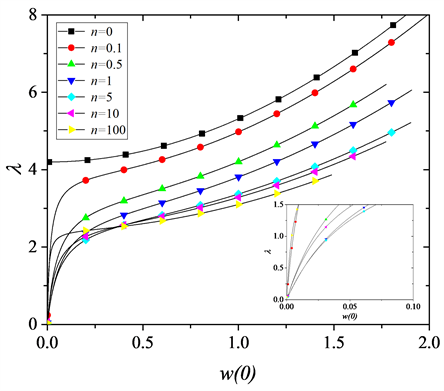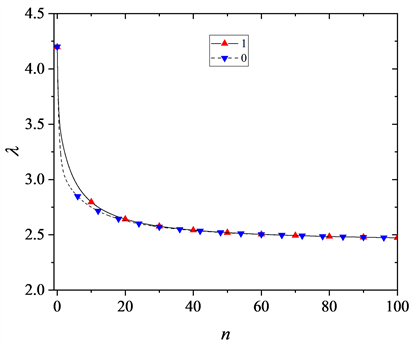3.2. 受热载荷作用

$u=w=0,{F}_{4}\left[\frac{\text{d}u}{\text{d}x}+\frac{1}{2}{\left(\frac{\text{d}w}{\text{d}x}\right)}^{2}\right]-\left(\frac{{\text{d}}^{2}w}{\text{d}{x}^{2}}+\frac{\nu }{x}\frac{\text{d}w}{\text{d}x}\right)-\stackrel{¯}{M}=0$ $x=1$ (18)

$\begin{array}{l}w=0,\left[\frac{\text{d}u}{\text{d}x}+\nu \frac{u}{x}+\frac{1}{2}{\left(\frac{\text{d}w}{\text{d}x}\right)}^{2}\right]-{F}_{1}\left(\frac{{\text{d}}^{2}w}{\text{d}{x}^{2}}+\frac{\nu }{x}\frac{\text{d}w}{\text{d}x}\right)-\stackrel{¯}{{N}_{0}}=0,\\ {F}_{4}\left[\frac{\text{d}u}{\text{d}x}+\nu \frac{u}{x}+\frac{1}{2}{\left(\frac{\text{d}w}{\text{d}x}\right)}^{2}\right]-\left(\frac{{\text{d}}^{2}w}{\text{d}{x}^{2}}+\frac{\nu }{x}\frac{\text{d}w}{\text{d}x}\right)-\stackrel{¯}{M}=0\end{array}$ $x=1$(19)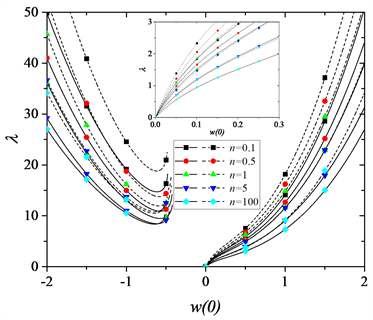(a) Tr = 0.1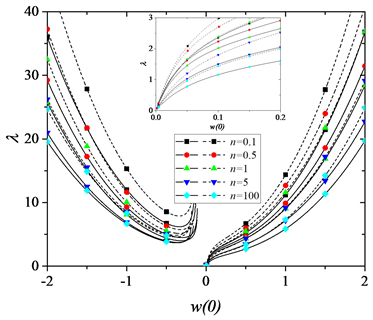(b) Tr = 0.5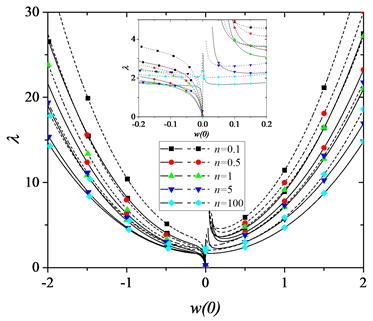(c) Tr = 1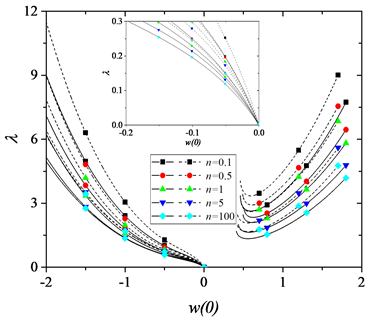(d) Tr = 5

Figure 3. Load deflection curve of FGM simply supported and immovable circular plate under non-uniform thermal loads for different values of temperature ratio Tr

$\frac{\text{d}}{\text{d}x}\left[\frac{1}{x}\frac{\text{d}}{\text{d}x}\left(x\stackrel{¯}{u}\right)\right]-{F}_{1}\frac{\text{d}}{\text{d}x}{\nabla }^{2}\stackrel{¯}{w}=0$ (20a)

${\nabla }^{4}\stackrel{¯}{w}+\stackrel{¯}{N}{\nabla }^{2}\stackrel{¯}{w}=0$ (20b)

$\stackrel{¯}{u}={F}_{1}\beta {A}_{0}\left({J}_{1}\left(\beta \right)x-{J}_{1}\left(\beta x\right)\right),\stackrel{¯}{w}={A}_{0}\left({J}_{0}\left(\beta x\right)-{J}_{0}\left(\beta \right)\right)$ (21)

$\begin{array}{l}\frac{{\text{d}}^{2}\Delta u}{\text{d}{x}^{2}}+\frac{1}{x}\frac{\text{d}\Delta u}{\text{d}x}-\frac{\Delta u}{{x}^{2}}-{F}_{1}\frac{{\text{d}}^{3}\Delta w}{\text{d}{x}^{3}}-\left({F}_{1}+x{A}_{0}\beta {J}_{1}\left(\beta x\right)\right)\frac{1}{x}\frac{{\text{d}}^{2}\Delta w}{\text{d}{x}^{2}}\\ +\left[{F}_{1}-{x}^{2}{A}_{0}\beta \left({J}_{0}\left(\beta x\right)-\frac{\nu }{x}{J}_{1}\left(\beta x\right)\right)\right]\frac{1}{{x}^{2}}\frac{\text{d}\Delta w}{\text{d}x}=0\end{array}$ (22a)

$\begin{array}{l}\frac{{\text{d}}^{4}\Delta w}{\text{d}{x}^{4}}+\frac{2}{x}\frac{{\text{d}}^{3}\Delta w}{d{x}^{3}}-\left[1+x\beta {A}_{0}{J}_{1}\left(\beta x\right)\left({F}_{1}{F}_{2}-2{F}_{3}\right)\left(1-\nu \right)+\frac{1}{2}{x}^{2}{A}_{0}^{2}{\beta }^{2}{\left({J}_{1}\left(\beta x\right)\right)}^{2}\right]\frac{1}{{x}^{2}}\frac{{\text{d}}^{2}\Delta w}{\text{d}{x}^{2}}\\ -\left[-{x}^{2}\beta {A}_{0}\left({J}_{0}\left(\beta x\right)\left({F}_{1}{F}_{2}-2{F}_{3}\right)-\left(1+\nu \right){F}_{1}{F}_{2}{J}_{1}\left(\beta \right)\right)\right]\frac{1}{{x}^{2}}\frac{{\text{d}}^{2}\Delta w}{\text{d}{x}^{2}}\\ +\left[1+x\beta {A}_{0}{J}_{1}\left(\beta x\right)\left({F}_{1}{F}_{2}-2{F}_{3}\right)\left(1-\nu \right)+\frac{3\nu }{2}{F}_{2}{x}^{2}{A}_{0}^{2}{\beta }^{2}{\left({J}_{1}\left(\beta x\right)\right)}^{2}\right]\frac{1}{{x}^{3}}\frac{\text{d}\Delta w}{\text{d}x}\\ +\left[-{x}^{2}\left(\left(1+\nu \right)\beta {A}_{0}{F}_{1}{F}_{2}{J}_{1}\left(\beta \right)-\nu \beta {A}_{0}{J}_{0}\left(\beta x\right)\left({F}_{1}{F}_{2}-2{F}_{3}\right)\right)\right]\frac{1}{{x}^{3}}\frac{\text{d}\Delta w}{\text{d}x}\\ -\left[{F}_{2}{A}_{0}^{2}{\beta }^{2}{J}_{1}\left(\beta x\right)\left({J}_{0}\left(\beta x\right)-\frac{1}{x}{J}_{1}\left(\beta x\right)\right)\right]\frac{\text{d}\Delta w}{\text{d}x}+\stackrel{¯}{N}\left(\frac{{\text{d}}^{2}\Delta w}{\text{d}{x}^{2}}+\frac{1}{x}\frac{\text{d}\Delta w}{\text{d}x}\right)\\ +\frac{{F}_{2}}{x}\beta {A}_{0}{J}_{1}\left(\beta x\right)\left(\nu \frac{\text{d}\Delta u}{\text{d}x}+\frac{\Delta u}{x}\right)+{F}_{2}\beta {A}_{0}\left[{J}_{0}\left(\beta x\right)-\frac{1}{x}{J}_{1}\left(\beta x\right)\right]\left(\frac{\text{d}\Delta u}{\text{d}x}+\frac{\nu }{x}\Delta u\right)=0\end{array}$ (22b)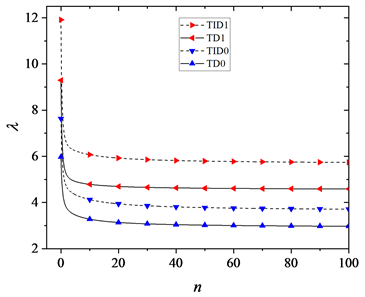(a) Tr = 0.1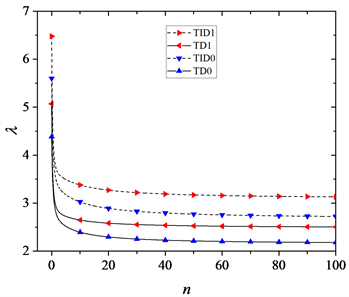(b) Tr = 0.5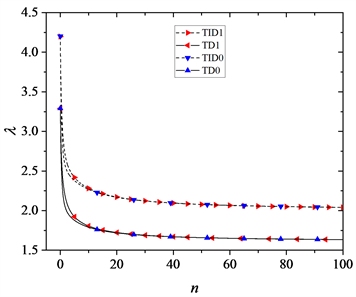(c) Tr = 1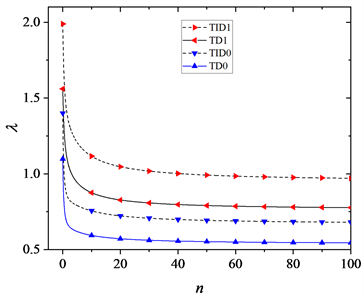(d) Tr = 5

Figure 4. Critical load vs. gradient of FGM simply supported and immovable circular plate under non-uniform thermal load for different values of temperature ratio Tr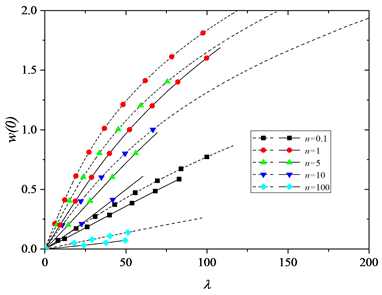Figure 5. Load deflection curve of FGM simply supported and movable circular plate under uniform thermal load

4. 结论

1) 机械载荷作用下，考虑前屈曲耦合变形时，FGM简支圆板的临界载荷增大。

2) 非均匀热载荷作用下，简支不可移边界下FGM圆板的前屈曲耦合变形使得临界载荷增大；而均温时，前屈曲耦合变形只在局部范围内对临界载荷有明显影响。

3) 材料的温度依赖性质降低了FGM圆板的临界载荷。

4) 均温下的简支可移FGM圆板的挠度随着热载荷的增加而增大，不出现“平台”，只是通常意义下的弯曲。

5) 陶瓷侧温度越来越低，金属侧温度越来越高时，FGM圆板的临界载荷越小。

6) 同一热载荷下，FGM圆板可能存在三种构形；随着上下面的温差变化，FGM圆板的构形会发生翻转。